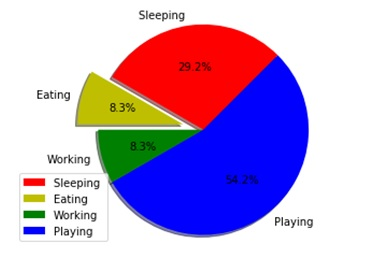# Python | Create pie-chat using matplotlib.pyplot

Here, we are implementing a python program to create a pie-chat using matplotlib.pyplot.
Submitted by Ayush Sharma, on November 25, 2018

Problem statement: Create pie-charts in python (using matplotlib.pyplot).

Program:

```import matplotlib.pyplot as plt

days = [1, 2, 3, 4, 5]
slices = [7,2,2,13]
cols = ['r','y','g','b']

my_labels = ["Sleeping ", "Eating", "Working", "Playing"]

plt.pie(slices,
labels=my_labels,
colors = cols,
startangle=45,
explode =(0,0.2,0,0),
autopct = '%1.1f%%')
plt.axis('equal')
plt.legend(loc=3)
plt.show()
```

OutputExplanation:

The pie function plots the pie chart. Mylabels attribute is given to the label of the pie function. Explode attribute is used to explode the part of the pie. There is also a shadow attribute in the pie function for giving a shadow effect. In legend function a loc variable is passed to specify the location of the legend.

Preparation